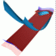Abstract and Contents
Next: 2. Overview of earlier methods
Previous: Abstract and Contents

# 1. Introduction

We consider a discrete dynamical system given by a diffeomorphism on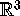. Such a map may be given explicitly, or be obtained as the Poincaré map of a vector field on a four-dimensional state space. Stable and unstable manifolds of invariant manifolds of saddle-type, here fixed points or invariant circles, play important roles in organizing the global dynamics. It is well-known that the stable manifolds form boundaries between different basins of attraction. Furthermore, the transverse intersection of stable and unstable manifolds leads to homoclinic or heteroclinic tangle, associated with chaos. These manifolds are global, often noncompact objects that can have very complicated structure. Only in special situations it is possible to find stable and unstable manifolds analytically. In general they need to be computed with numerical methods.

In this paper we compute the two-dimensional unstable manifold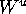of an invariant manifold of saddle-type of a three-dimensional diffeomorphism. (The stable manifold can be computed by considering the inverse.) There are two different cases: the unstable manifoldof a hyperbolic fixed point, and the unstable manifold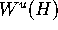of a normally hyperbolic invariant circle H. The Unstable Manifold Theorem [Hirsch et al. 1977, Palis and De Melo 1982] guarantees the existence of the local unstable manifold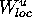in a neighborhood of the invariant manifold of saddle-type. The two cases are sketched in Figure 1. See also the animations.

The idea is to obtain the global unstable manifoldby globalizing the local unstable manifold. In practice, we reliably compute a sufficient piece of, so that conclusions on the global dynamics can be drawn. The unstable manifold is represented by a discrete set of mesh points. The quality of the mesh can be prescribed, and the mesh is adapted whenever necessary. Our algorithm was originally developed for the computation of unstable manifolds of a normally hyperbolic invariant circle of saddle-type. With a slight adaptation, it can also be used to find a two-dimensional unstable manifold of a hyperbolic fixed point.

As starting data for our algorithm we need an approximation of the local unstable manifold. There are different algorithms for computingof a hyperbolic fixed point; see [Osinga 1996] and biblio therein. The work presented here was motivated by the possibility of obtaining the starting data for the computation of the unstable manifoldof an invariant circle H of saddle-type in the form of a linear approximation of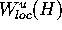with the method in [Osinga 1996, Broer et al. 1996, Broer et al. 1997]. Their method is a variation of the graph transform that allows the computation of a normally hyperbolic invariant circle of saddle-type of a three-dimensional map. The key idea is to start with a known invariant circle H of a map f together with the Df-invariant splitting of the tangent space at H. This splitting induces Df-invariant stable and unstable normal bundles that are embedded inin a neighborhood of H. Because H is normally hyperbolic, these embedded normal bundels form a well-defined coordinate system in a neighborhood of H. The invariant circle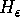of a small perturbationof f is computed as the graph over the known circle H in the coordinate system given by the embedded normal bundles. As a special feature of the method the new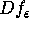-invariant splitting of the invariant circleis computed in a second step, regardless of the dynamics on. Consequently, the method can be used in a continuation setting: a known invariant circle can be followed by increasing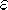in small steps. The embedded unstable normal bundleof [Osinga 1996, Broer et al. 1996, Broer et al. 1997] is the first order approximation of, the local unstable manifold of H.

This allows us to globalize this first order approximation to compute a significant piece of. To be more concrete, the invariant circle H is known in a finite mesh M of points, and at each mesh pointwe are given the normal directionof the embedded normal bundle. (Since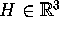is of saddle-type,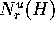is a vector.) We now choose a linear foliation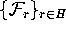of the state space so that each leaf has a unique intersection with H. From the unstable normal bundle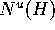above we compute unit vectors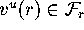for all, such that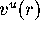is tangent to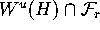. Starting from the linear approximation given by M and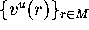, we compute the intersection of the unstable manifoldwith the finitely many leaves of. For this to work we need the following.

Foliation Condition
In each leaf ofthere is a unique curve of intersection with the unstable manifold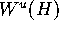. In other words, the unstable manifold intersects each leaf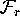transversally.

Assuming this foliation condition is satisfied, we can compute the unstable manifold in each of the leaves of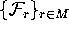as a sequence of points that have a prescribed distance from each other. The set of sequences in a finite number of leaves defines a mesh that represents the unstable manifold. This computation can be done in steps by adding rings or bands, that is, by adding a single new point to the sequence in each leaf. In this way, one can see the unstable manifold grow during the computation.

The linear foliation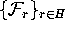has no dynamical property, but should be seen as an a priori definition of the mesh. By adding additional leaves during the computation we guarantee the quality of the mesh on the unstable manifold. By construction, our method is independent of the dynamics on the invariant circle. We can use it for the computation of the two-dimensional unstable manifold of a fixed point, if we interpret the fixed point as an invariant circle in polar coordinates. A detailed description of the algorithm can be found in Section 3.

The procedure of adding rings or bands fails when the computed portion of the unstable manifold no longer intersects each leaf ofin a unique curve. A piece of the intersection is then missed in the computation, and the algorithm is lacking information about a part of the unstable manifold. Because this information is necessary in the computation, the algorithm stops. This is discussed in more detail in Section 6; see also Figure 6 and Figure 18. Note that by definition the foliationis transverse toso that at least a part ofcan be computed. Furthermore, we think that many interesting examples satisfy the foliation condition; see Section 5. Possible relaxations of the foliation condition are discussed in Section 6.

In summary, we present an algorithm that computes a growing piece of the two-dimensional unstable manifold of a normally hyperbolic invariant circle of saddle-type or a hyperbolic saddle point, until the unstable manifold becomes tangent to the foliation. The mesh representing the invariant manifold is of a prescribed quality. This allows us to study the global dynamics of many interesting systems, in particular those that satisfy the Foliation Condition.

This paper is organized as follows. In Section 2 we give an overview over the literature on the calculation of global unstable manifolds. In Section 3 we give a detailed description of the algorithm, and Section 4 deals with its correctness. The performance of our method is demonstrated in Section 5 with a number of examples. The applicability of the algorithm and some open problems are discussed in Section 6.

Next: 2. Overview of other methods
Previous: Abstract and ContentsAbstract and Contents

Written by: Bernd Krauskopf & Hinke Osinga
Created: May 27 1997 --- Last modified: Wed Jul 2 10:50:50 1997